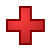Games
Problems
Go Pro!

# Addition by 2

Quizzes > Mathematics > Arithmetic > AdditionPractice your addition facts with this simple math quiz from The Problem Site.

When you click Start Quiz, multiple-choice questions will appear one at a time. Choose the correct answers, and find out how much you know!
Quiz by Mr. TwitchellAssign this quiz
Click here to assign this quiz to your students.# Understanding Coronavirus Spread

A Question and Answer session with Professor Puzzler about the math behind infection spread.

# Blogs on This SiteReviews and book lists - books we love!The site administrator fields questions from visitors.

# Questions

Here are some of the questions you may encounter in the quiz Addition by 2.

2 + 4 = ?
2 + 6 = ?
2 + 1 = ?
2 + 7 = ?
2 + 8 = ?
2 + 2 = ?
2 + 0 = ?
2 + 3 = ?
2 + 9 = ?
2 + 5 = ?Like us on Facebook to get updates about new resources